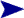Calculators for Statistical Table Entries

Calculators for Statistical Table Entriesz to Pchi-square to Pt to Pr to PF to PFisher r-to-z transformation.05 and .01 critical values of the Studentized range statistic QOdds & Log Odds

z to P Calculator
For any given value of z, this section will calculate
 º the respective one-tailed probabilities of —z and +z; º the two-tailed probability of±z; º and the proportion of the normal distribution falling between —z and +z.

To proceed, enter the value of z in the designated cell and click «Calculate». Note that the programming for this section is able to perform meaningful probability calculations only for cases where |z|<5.Click here to see the details of the sampling distribution to which any particular value of z belongs.
 z =

 Probabilities P Text will appear in this box only if the value of |z| is greater than 3.75. one-tailed for —z one-tailed for +z two-tailed for ±z area between ±z

Chi-Square to P CalculatorFor values of df between 1 and 20, inclusive, this section will calculate the proportion of the relevant sampling distribution that falls to the right of a particular value of chi-square. To proceed, enter the values of chi-square and df in the designated cells and click «Calculate».
 Chi-Square df P
distribution to which any particular value of
chi-square belongs. At the prompt, enter the
appropriate value of df.

t to P CalculatorThis section will calculate the one-tail and two-tail probabilities of t for any given value of df. To proceed, enter the values of t and df in the designated cells and click «Calculate».
 t df
 P one-tailed two-tailed
distribution to which any particular value of t
belongs. At the prompt, enter the appropriate

r to P Calculator
If the true correlation between X and Y within the general population is rho=0, and if the size of the sample, N, on which an observed value of r is based is equal to or greater than 6, then the quantity

 t = rsqrt[(1—r2)/(N—2)]

is distributed approximately as t with df=N2. Application of this formula to any particular observed sample value of r will accordingly test the null hypothesis that the observed value comes from a population in which rho=0.

To assess the significance of any particular instance of r, enter the values of N[>6] and r into the designated cells below, then click the 'Calculate' button.
 N = r = t df
 P one-tailed two-tailed

Fisher r-to-z Transformation

For any particular value of r, the Pearson product-moment correlation coefficient, this section will perform the Fisher r-to-z transformation according to the formula
 zr = (1/2)[loge(1+r) - loge(1-r)]
If a value of n is entered (optional), it will also calculate the standard error of zr as
 SEzr = 1/sqrt[n-3]

 r = n = zr = SEzr =

F to P CalculatorThis section will calculate the proportion of the relevant sampling distribution that falls to the right of a particular value of F, as defined by the degrees of freedom for the numerator and denominator of the F-ratio. To proceed, enter the values of F and df in the designated cells and click «Calculate».

 F Click here to see the details of the sampling distribution to which any particular value of F belongs. At the prompt, enter the df values for numerator and denominator. df numerator denominator P

Critical Values of Q
This section will calculate the .05 and .01 critical values for the Studentized range statistic Q. To proceed, enter the number of groups in the analysis (k) and the number of degrees of freedom, and then click «Calculate». Note that the value of k must be between 3 and 10, inclusive.

 k df Q.05 Q.01

Odds & Log Odds
Given p, an observed proportion or probability:Odds = p/(1-p)Log Odds: LO = loge[Odds]= loge[p/(1-p)]
Given the Log Odds:Odds = exp[LO]
Given the Odds:p = Odds/(1+Odds)

Enter any one of these items—p, Odds, or Log Odds into the designated cell, then click the «Calculate» button to fetch the corresponding values of the other two. If entering p, the entry can be either a decimal fraction or a common fraction.

 p Odds Log Odds